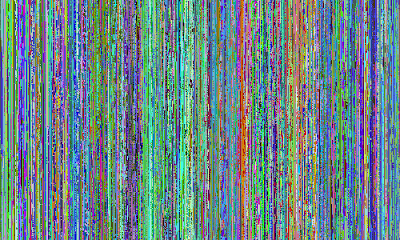## December 17, 2008

### Visualizing Server Access Logs

While playing around with PDL and Apache access logs I realized that IP addresses could very easily be converted into an image. The first three octects of the address can be converted to RGB values of a pixel, since both range from 0 to 255. The result looks something like this:And here's the Perl code that parses the log for IP addresses, and then creates the image, using PDL.
```use PDL;
use PDL::NiceSlice;
use PDL::IO::Pic;

my \$file = "input.log";

my \$w= 500;
my \$h = 300;//create a piddle (matrix)my \$pic = zeroes(3,\$w,\$h);open (LOG,"<\$file") || die "can't open log file";   my \$ct =0; while(<LOG>) {
if(/^(\d+).(\d+).(\d+).(\d+)/){
\$col = floor(\$ct/\$h);
\$row = \$ct - (floor(\$ct/\$h) *\$h);
\$pic(0:0,\$col:\$col,\$row:\$row) .=\$1;
\$pic(1:1,\$col:\$col,\$row:\$row) .=\$2;
\$pic(2:2,\$col:\$col,\$row:\$row) .=\$3;
\$ct ++;
if(\$ct > (\$w * \$h) -1){
print "done\n";
last;
}
}
}
close LOG;
\$pic->wpic("output.tif");
```